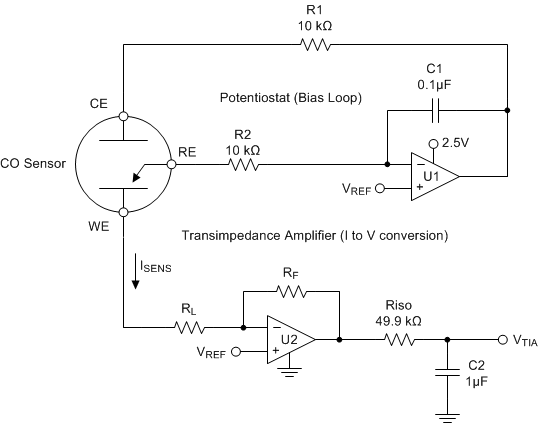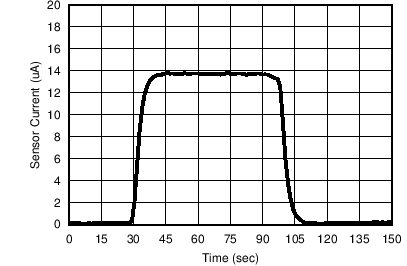SNOSD34A August   2016  – November 2016

PRODUCTION DATA.

1. Features
2. Applications
3. Description
4. Revision History
5. Pin Configuration and Functions
6. Specifications
7. Detailed Description
8. Application and Implementation
9. Power Supply Recommendations
10. 10Layout
11. 11Device and Documentation Support
12. 12Mechanical, Packaging, and Orderable Information

• DGK|8
• DGK|8

## 8 Application and Implementation

NOTE

Information in the following applications sections is not part of the TI component specification, and TI does not warrant its accuracy or completeness. TI’s customers are responsible for determining suitability of components for their purposes. Customers should validate and test their design implementation to confirm system functionality.

### 8.1 Application Information

The TLV880x is a ultra-low power operational amplifier that provides 6 kHz bandwidth with only 320nA typical quiescent current, and near precision drift specifications. These rail-to-rail output amplifiers are specifically designed for battery-powered applications. The input common-mode voltage range extends to the negative supply rail and the output swings to within millivolts of the rails, maintaining a wide dynamic range.

### 8.2 Typical Application: Three Terminal CO Gas Sensor AmplifierFigure 38. Three Terminal Gas Sensor Amplifer Schematic

#### 8.2.1 Design Requirements

Figure 38 shows a simple micropower potentiostat circuit for use with three terminal unbiased CO sensors, though it is applicable to many other type of three terminal gas sensors or electrochemical cells.

The basic sensor has three electrodes; The Sense or Working Electrode (“WE”), Counter Electrode (“CE”) and Reference Electrode (“RE”). A current flows between the CE and WE proportional to the detected concentration.

The RE monitors the potential of the internal reference point. For an unbiased sensor, the WE and RE electrodes must be maintained at the same potential by adjusting the bias on CE. Through the Potentiostat circuit formed by U1, the servo feedback action will maintain the RE pin at a potential set by VREF.

R1 is to maintain stability due to the large capacitance of the sensor. C1 and R2 form the Potentiostat integrator and set the feedback time constant.

U2 forms a transimpedance amplifier ("TIA") to convert the resulting sensor current into a proportional voltage. The transimpedance gain, and resulting sensitivity, is set by RF according to Equation 2.

Equation 2. VTIA = (-I * RF) + VREF

RL is a load resistor of which the value is normally specified by the sensor manufacturer (typically 10 ohms). The potential at WE is set by the applied VREF. Riso provides capacitive isolation and, combined with C2, form the output filter and ADC reservoir capacitor to drive the ADC.

#### 8.2.2 Detailed Design Procedure

For this example, we will be using a CO sensor with a sensitivity of 69nA/ppm. The supply votlage and maximum ADC input voltage is 2.5V, and the maximum concentration is 300ppm.

First the VREF voltage must be determined. This voltage is a compromise between maximum headroom and resolution, as well as allowance for "footroom" for the minimum swing on the CE terminal, since the CE terminal generally goes negative in relation to the RE potential as the concentration (sensor current) increases. Bench measurements found the difference between CE and RE to be 180mV at 300ppm for this particular sensor.

To allow for negative CE swing "footroom" and voltage drop across the 10k resistor, 300mV was chosen for VREF.

Therefore +300mV will be used as the minimum VZERO to add some headroom.

Equation 3. VZERO = VREF = +300mV

where

• VZERO is the zero concentration voltage
• VREF is the reference voltage (300mV)

Next we calculate the maximum sensor current at highest expected concentration:

Equation 4. ISENSMAX = IPERPPM * ppmMAX = 69nA * 300ppm = 20.7uA

where

• ISENSMAX is the maximum expected sensor current
• IPERPPM is the manufacturer specified sensor current in Amps per ppm
• ppmMAX is the maximum required ppm reading

Now find the available output swing range above the reference voltage available for the measurement:

Equation 5. VSWING = VOUTMAX – VZERO = 2.5V – 0.3V = 2.2V

where

• VSWING is the expected change in output voltage
• VOUTMAX is the maximum amplifer output swing (usually near V+)

Now we calculate the transimpedance resistor (RF) value using the maximum swing and the maximum sensor current:

Equation 6. RF = VSWING / ISENSMAX = 2.2V / 20.7µA = 106.28 kΩ (we will use 110 kΩ for a common value)

#### 8.2.3 Application CurveFigure 39. Monitored Voltages when exposed to 200ppm CO

Figure 39 shows the resulting circuit voltages when the sensor was exposed to 200ppm step of carbon monoxide gas. VC is the monitored CE pin voltage and clearly shows the expected CE voltage dropping below the WE voltage, VW, as the concentration increases.

VTIA is the output of the transimpedance amplifer U2. VDIFF is the calculated difference between VREF and VTIA, which will be used for the ppm calculation.Figure 40. Calculated Sensor CurrentFigure 41. Calculated ppm

Figure 40 shows the calculated sensor current using the formula in Equation 7 :

Equation 7. ISENSOR = VDIFF / RF = 1.52V / 110 kΩ = 13.8uA

Equation 8 shows the resulting conversion of the sensor current into ppm.

Equation 8. ppm = ISENSOR / IPERPPM = 13.8µA / 69nA = 200

Total supply current for the amplifier section is less than 700 nA, minus sensor current. Note that the sensor current is sourced from the amplifier output, which in turn comes from the amplifier supply voltage. Therefore, any continuous sensor current must also be included in supply current budget calculations.

### 8.3 Do's and Don'ts

Do properly bypass the power supplies.

Do add series resistance to the output when driving capacitive loads, particularly cables, Muxes and ADC inputs.

Do add series current limiting resistors and external schottky clamp diodes if input voltage is expected to exceed the supplies. Limit the current to 1mA or less (1KΩ per volt).